### Constraint Data Set

The Constraint data set defines linear constraints, variable types, bounds on variable domains, and an objective function. You can use a Constraint data set in lieu of, or in combination with, a LINCON or a VARIABLE statement (or both) in order to define linear constraints, variable types, and variable bounds. You can use the Constraint data set in lieu of, or in combination with, the OBJ statement to specify an objective function. The Constraint data set is specified by using the CONDATA= option in the PROC CLP statement.

The Constraint data set must be in dense input format. In this format, a model’s columns appear as variables in the input data set and the data set must contain the _TYPE_ variable, at least one numeric variable, and any reserved variables. Currently, the only reserved variable is the _RHS_ variable. If this requirement is not met, the CLP procedure terminates. The _TYPE_ variable is a character variable that tells the CLP procedure how to interpret each observation. The CLP procedure recognizes the following keywords as valid values for the _TYPE_ variable: EQ, LE, GE, NE, LT, GT, LOWERBD, UPPERBD, BINARY, FIXED, MAX, and MIN. An optional character variable, _ID_, can be used to name each row in the Constraint data set.

#### Linear Constraints

For the _TYPE_ values EQ, LE, GE, NE, LT, and GT, the corresponding observation is interpreted as a linear constraint. The _RHS_ variable is a numeric variable that contains the right-hand-side coefficient of the linear constraint. Any numeric variable other than _RHS_ that appears in a VARIABLE statement is interpreted as a structural variable for the linear constraint.

The _TYPE_ values are defined as follows:

EQ (=)

defines a linear equality of the form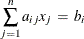LE (<=)

defines a linear inequality of the form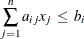GE (>=)

defines a linear inequality of the form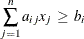NE (<>)

defines a linear disequation of the form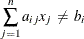LT (<)

defines a linear inequality of the form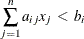GT (>)

defines a linear inequality of the form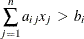#### Domain Bounds

The keywords LOWERBD and UPPERBD specify additional lower bounds and upper bounds, respectively, on the variable domains. In an observation where the _TYPE_ variable is equal to LOWERBD, a nonmissing value for a decision variable is considered to be a lower bound for that variable. Similarly, in an observation where the _TYPE_ variable is equal to UPPERBD, a nonmissing value for a decision variable is considered to be an upper bound for that variable. Note that lower and upper bounds defined in the Constraint data set are overridden by lower and upper bounds that are defined by using a VARIABLE statement.

#### Variable Types

The keywords BINARY and FIXED specify numeric variable types. If the value of _TYPE_ is BINARY for an observation, then any decision variable with a nonmissing entry for the observation is interpreted as being a binary variable with domain {0,1}. If the value of _TYPE_ is FIXED for an observation, then any decision variable with a nonmissing entry for the observation is interpreted as being assigned to that nonmissing value. In other words, if the value of the variable X isin an observation for which _TYPE_ is FIXED, then the domain of X is considered to be the singleton {}. The valueshould belong to the domain of X, or the problem is deemed infeasible.

#### Objective Function

The keywords MAX and MIN specify the objective function of a maximization or a minimization problem, respectively. In an observation where the _TYPE_ variable is equal to MAX or MIN, a nonmissing value for a decision variable is the coefficient of this variable in the objective function. The value specified for _RHS_ is ignored in this case.

The bisection method is used to find the optimal objective value within the specified or derived lower and upper bounds. A solution is considered optimal if the difference between consecutive objective values is less than or equal to the tolerance. You can use the OBJ statement to specify the tolerance in addition to upper and lower bounds on the objective value.

When an optimal solution is found, the solution is stored in the output data set and the resulting objective value is stored in the macro variable _ORCLP_. The objective value is not necessarily optimal when it is computed within a time limit specified by the MAXTIME= option. In this case, the last valid solution computed within the time limit appears in the output data set. See the macro variable _ORCLP_ for more information about solution status.

The MAX and MIN functions are defined as follows:

MAX

defines an objective function of the form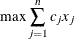MIN

defines an objective function of the form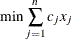#### Variables in the CONDATA= Data Set

Table 3.3 lists all the variables that are associated with the Constraint data set and their interpretations by the CLP procedure. For each variable, the table also lists its type (C for character, N for numeric), the possible values it can assume, and its default value.

Table 3.3: Constraint Data Set Variables

Name

Type

Description

Allowed Values

Default

_TYPE_

C

Observation type

EQ, LE, GE, NE, LT, GT, LOWERBD, UPPERBD, BINARY, FIXED, MAX, MIN

_RHS_

N

Right-hand-side coefficient

0

_ID_

C

Observation name (optional)

Any numeric variable other than _RHS_

N

Structural variable